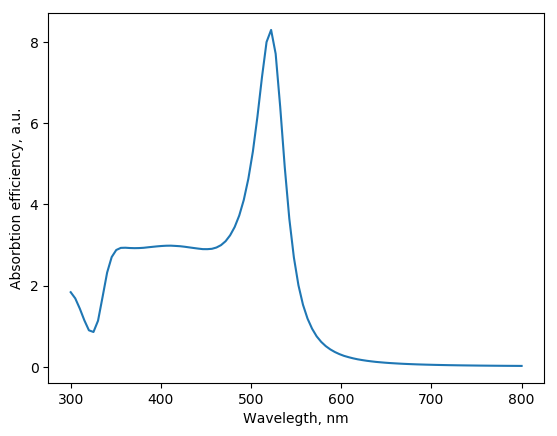# Spheres setup¶

MSTM code requires explicit setup of the positons and sizes of spheres. There are several classes designed in MSTM-studio to help this setup.

## Example: particles aggregate¶

Script to construct spheres with random sizes placed on a regular grid:

from mstm_studio.mstm_spectrum import LogNormalSpheres
from mstm_studio.alloy_AuAg import AlloyAuAg

spheres = LogNormalSpheres(9, 10.0, 0.2, 5., AlloyAuAg(1.))
while spheres.check_overlap():
print('spheres are overlapping, regenerating...')
spheres = LogNormalSpheres(9, 10.0, 0.2, 5., AlloyAuAg(1.))
print(spheres.a)


Sample output:

Box size estimated as: 77.0 nm
Desired number of particles: 9
Number of particles in a box: 8
Resulted number of particles: 8
spheres are overlapping, regenerating...
Box size estimated as: 77.0 nm
Desired number of particles: 9
Number of particles in a box: 8
Resulted number of particles: 8
[ 9.307773    8.61185299  9.92867988  8.84140858  9.87175352  8.71090184
9.71505038 12.40459688]


## Classes¶

class mstm_studio.mstm_spectrum.Spheres[source]

Abstract collection of spheres

Object fields:
N: int
number of spheres
x, y, z: numpy arrays
coordinates of spheres centers
a: list or arrray
materials: numpy array
Material objects or strings

Creates empty collection of spheres. Use child classes for non-empty!

append(sphere)[source]

Append by data from SingleSphere object

Parameter:

sphere: SingleSphere
check_overlap(eps=0.001)[source]

Check if spheres are overlapping

delete(i)[source]

Delete element with index i

extend(spheres)[source]

Append by all items from object spheres

get_center(method='')[source]

calculate center of masses in assumption of uniform density

Parameter:

method: string {‘’|’mass’}
If method == ‘mass’ then center of masses (strictly speaking, volumes) is calculated. Otherwise all spheres are averaged evenly.
load(filename, mat_filename='etaGold.txt', units='nm')[source]

Parameters:

filename: string
mat_filename: string
all spheres will have this material (sphere-material storaging is not yet implemented)
units: string {‘mum’|’nm’}
distance units. If ‘mum’ then coordinated will be scaled (x1000)
save(filename)[source]

Saves spheres coordinates and radii to file.

Parameter:

filename: string
class mstm_studio.mstm_spectrum.SingleSphere(x, y, z, a, mat_filename='etaGold.txt')[source]

Collection of spheres with only one sphere

Parameters:

x, y, z: float
coordinates of spheres centers
a: float
mat_filename: string, float, complex value or Material object
material specification
class mstm_studio.mstm_spectrum.ExplicitSpheres(N=0, Xc=[], Yc=[], Zc=[], a=[], mat_filename='etaGold.txt')[source]

Create explicitely defined spheres

Parameters:
N: int
number of spheres
Xc, Yc, Zc: lists or numpy arrays
coordinates of the spheres centers
a: list or numpy array
mat_filename: string, list of strings, Material or list of
Materials specification of spheres material

Note: If only first array Xc is supplied, than all data is assumed zipped in it, i.e.: Xc = [X1, Y1, Z1, a1, …, XN, YN, ZN, aN]

class mstm_studio.mstm_spectrum.LogNormalSpheres(N, mu, sigma, d, mat_filename='etaGold.txt')[source]

The set of spheres positioned on the regular mesh with random Log-Normal distributed sizes. In the case overlapping of the spheres the sizes should(?) be regenerated.

Parameters:

N: int
number of spheres
mu, sigma: floats
parameters of Log-Normal distribution
d: float
average empty space between spheres centers
mat_filename: string or Material object
specification of spheres material

# MSTM run¶

T-matrix formalism prposed by Waterman [Khlebtsov2013] is one of the generalization of Mie theory towards the multiple spherical targets. The Multi Sphere T-matrix (MSTM) Fortran code is developed by Mischnko and Mackowsky [Mackowski2011]. The SPR class implements functionality required for extinction spectra calculation in visible range. Note, that Fortran code have wider functionality, including near field calculations, angle-dependent calculations, etc, which are not currently implemented. Consult the MSTM website <http://eng.auburn.edu/users/dmckwski/scatcodes/> for details.

## Example: core-shell particle¶

Absorbtion efficiency (normalized cross-section) of gold-silver core-shell particle.

from mstm_studio.alloy_AuAg import AlloyAuAg
from mstm_studio.mstm_spectrum import SPR, ExplicitSpheres
import matplotlib.pyplot as plt
import numpy as np

wls = np.linspace(300, 800, 100)    # define wavelengths
spr = SPR(wls)                      # create SPR object
spr.environment_material = 'glass'  # set matrix

spheres = ExplicitSpheres(2, [0,0,0,10,0,0,0,12], mat_filename=[AlloyAuAg(1.),AlloyAuAg(0.)])
spr.set_spheres(spheres)
spr.set_incident_field(fixed=False)
spr.simulate()

plt.plot(spr.wavelengths, spr.absorbtion)
plt.xlabel('Wavelegth, nm')
plt.ylabel('Absorbtion efficiency, a.u.')
plt.savefig('core-shell_mstm.png', bbox_inches='tight')## Class¶

class mstm_studio.mstm_spectrum.SPR(wavelengths)[source]

Class for calculation of surface plasmin resonance (SPR), running MSTM external code. The MSTM executable should be set in MSTM_BIN environment variable. Default is ~/bin/mstm.x

Parameter:
wavelengths: numpy array
Wavelegths in nm
plot()[source]

Plot results with matplotlib.pyplot

set_incident_field(fixed=False, azimuth_angle=0.0, polar_angle=0.0, polarization_angle=0.0)[source]

Set incident wave orientation and polarization

Parameters:

fixed: bool
True - fixed orientation and polarized light False - average over all orientations and polarizations

azimuth_angle, polar_angle: float (degrees)

polarization_angle: float (degrees)
!sensible only for near field calculation! polarization angle relative to the k-z palne. 0 - X-polarized, 90 - Y-polarized (if azimuth and polar angles are zero).
simulate(outfn=None)[source]

Start the simulation.

The inpuit parameters are read from object dictionary paramDict. Routine will prepare input file scriptParams.inp in the temporary folder, which will be deleted after calculation.

After calculation the result depends on the polarization setting. For polarized light the object fields will be filled:

extinction_par, extinction_ort, absorbtion_par, absorbtion_ort, scattering_par, scattering_ort.

While for orientation-averaged calculation just:

extinction, absorbtion and scattering.
write(filename)[source]

Save results to file

 [Khlebtsov2013] Khlebtsov, “T-matrix method in plasmonics: An overview” J. Quant. Spectrosc. Radiat. Transfer (2013) 123, 184-217, Peter C. Waterman and his scientific legacy
 [Mackowski2011] Mackowski, M. Mishchenko,”A Multiple Sphere T-matrix Fortran Code for Use on Parallel Computer Clusters” J. Quant. Spectrosc. Radiat. Transfer (2011) 112, 2182–2192